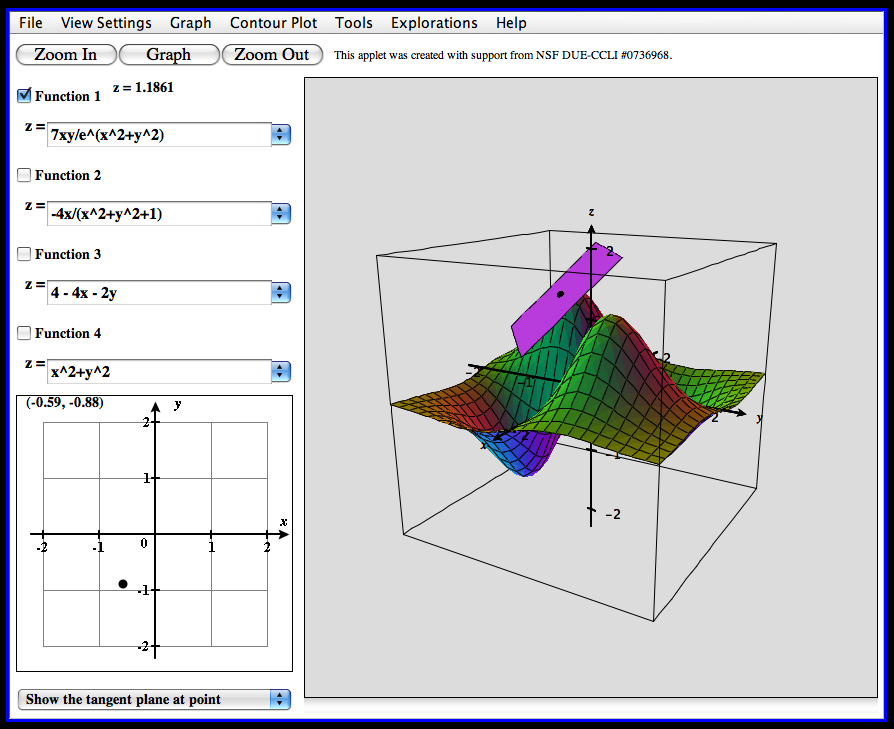### Multivariable Calculus SP 2020-21 MAT-2430-S00

This course is an introduction to the calculus of functions in more than one variable. Students explore topics including vector algebra, lines and planes, partial derivatives, the gradient, graphing in three dimensions, multiple integrals, vector integral calculus, and Stokes? and Divergence Theorems. Graphing calculators, DERIVE, and MAPLE may be used extensively to explore and reinforce the material.Prerequisite: MAT 2420 Calculus II or equivalent.